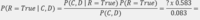# Fair Coin and Fair Dice probability

#### kubren

##### New member
Exercise: You will win if both a fair coin lands heads AND a fair die lands 6. After the coin is flipped and the die is rolled you ask if at least one of these events has occurred and you are told "yes."

One of the question is about specifying and calculating the posterior distribution for the joint probability of the coin and dice events given the event that the coin is heads or dice is six, 𝑃(𝐶,𝐷|𝑅 = 𝑇𝑟𝑢𝑒).

Using Bayes theorem, this is what I have managed to achieve so far:

• p(heads and six) = 1/12 or (0.083)
• p(heads or six) = 7/12 or (0.583)
• P(R=True): Prior probability
• P(C, D): Evidence
• P(𝑅 = 𝑇𝑟𝑢𝑒| 𝐶,𝐷): Likelihood
• P(𝐶,𝐷|𝑅 = 𝑇𝑟𝑢𝑒): Posterior probabilityI don't know what value the likelihood should be. Also, I would like to know if im following the correct approach?

#### Attachments

• 7.7 KB Views: 2

#### Dr.Peterson

##### Elite Member
What events are you calling R, C, and D? Also, in your notation, what does a comma mean?

#### kubren

##### New member
What events are you calling R, C, and D? Also, in your notation, what does a comma mean?
Hi,

The variables are as follows:

D = Dice (6)

The comma is set intersection like the probability of C and D given R (heads or 6).

#### Dr.Peterson

##### Elite Member
Hi,

The variables are as follows:

D = Dice (6)

The comma is set intersection like the probability of C and D given R (heads or 6).
So to finish your equation and solve for "?", you want to know P(Head or Six | Head and Six). That seems pretty simple to me when it's written out like that ...

That is true; but I would probably have used either a version of Bayes Theorem that directly gives the goal, P(Head and Six | Head or Six), such as is given here, or just use the definition of conditional probability.

•kubren

#### kubren

##### New member
So to finish your equation and solve for "?", you want to know P(Head or Six | Head and Six). That seems pretty simple to me when it's written out like that ...

That is true; but I would probably have used either a version of Bayes Theorem that directly gives the goal, P(Head and Six | Head or Six), such as is given here, or just use the definition of conditional probability.
Thank you for getting back to me. Iam confused as to what value P(Head and Six | Head or Six) should be? Would it be 7/12 +1/12.

#### tkhunny

##### Moderator
Staff member
 Heads AND Six 1​ 2​ 3​ 4​ 5​ 6​ Heads X Tails Heads OR Six 1​ 2​ 3​ 4​ 5​ 6​ Heads X X X X X X Tails X

•kubren

#### Dr.Peterson

##### Elite Member
Thank you for getting back to me. I am confused as to what value P(Head and Six | Head or Six) should be? Would it be 7/12 +1/12.
No, that is how you would combine mutually exclusive events with an "or". It has nothing to do with this problem.

Think about the meaning of "P(Head or Six | Head and Six)". It means the probability that the coin is a head OR the die is a 6, given that the coin is a head AND the die is a 6. Do you see that that is 1?

Now, what you are ultimately looking for is P(Head and Six | Head or Six). That can be found by solving the equation you wrote, or by applying the formula in the source I provided; or by tkhunny's demonstration of what the events (Head and Six) and (Head or Six) themselves are. What fraction of the latter are in the former? That will be your answer. (I like this approach more than formulas, because it relates more closely to what things actually mean.)

•kubren

#### HallsofIvy

##### Elite Member
There are a total of 2*6= 12 distinct results possible when you "flip a coin and roll a die". In six of those the coin landed "heads" and in 2 of them the die came up "6". But one of those were "heads and 6". Since we don't want to count that twice, there were 6+ 2- 1= 7 distinct situations in which we had either a head or a 6 or both. One of those 7 was "both a head and a 6".

•kubren

#### kubren

##### New member
Thank you all for taking the time to explain this. It all makes sense now.